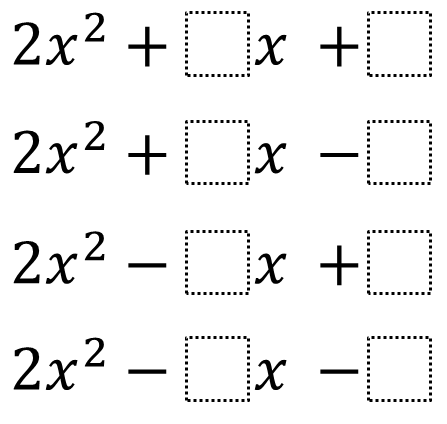Directions: Using the digits 1 to 9 at most one time each, place a digit in each box to construct four different quadratic expressions that can be factored as two binomials with integer coefficients and terms.### Hint

Try starting with a random c (constant term), and use this to determine b (first degree coefficient).

2x^2+3x+1 = (2x+1)(x+1)
2x^2-4x+2 = (2x-2)(x-1)
2x^2+9x-5 = (2x-1)(x+5)
2x^2-6x-8 = (2x+2)(x-4)

(Others are possible)

Source: Giles Fox